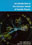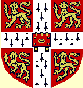© CAMBRIDGE UNIVERSITY PRESS 1998

1.5 Spectroscopy of Systems of Light Quarks

As will be discussed in Chapter 16, the masses of the u and d quarks are quite small, of the order of a few MeV / c2, closer to the electron mass than to a meson or baryon mass. A u or d quark confined within a distance1 fm has, by the uncertainty principle, a momentum p/ (1 fm)200 MeV / c, and hence its energy is Epc200 MeV, almost independent of the quark mass. All quarks have the same strong interactions. As a consequence the physics of light quark systems is almost independent of the quark masses. There is an approximate SU (2) isospin symmetry (Section 16.6), which is evident in the Standard Model.

The symmetry is not exact because of the different quark masses and different quark charges. The symmetry breaking due to quark mass differences prevails over the electromagnetic. In all cases where two particles differ only in that a d quark is substituted for a d quark, the particle with the d quark is more massive. For example, the neutron is more massive than the proton, even though the mass, ~ 2 MeV / c2, associated with the electrical energy of the charged proton is far greater than that associated with the (overall neutral) charge distribution of the neutron. We conclude that the d quark is heavier than the u quark.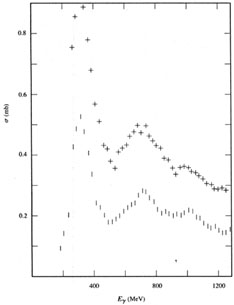Figure 1.1. The photon cross-section for hadron production by photons on protons (dashes) and deuterons (crosses). The difference between these cross-sections is approximately the cross-section for hadron production by photons on neutrons. (After Armstrong et al. (1972).)

The evidence for the existence of quarks came first from nucleon spectroscopy. The proton and neutron have many excited states which appear as resonances in photon-nucleon scattering and in pion-nucleon scattering (Fig. 1.1). Hadron states containing light quarks can be classified using the concept of isospin. The u and d quarks are regarded as a doublet of states | u> and | d>, with I = 1/2 and I3 = + 1/2, - 1/2, respectively. The total isospin of a baryon made up of three u or d quarks is then I = 3/2 or I = 1/2. The isospin 3/2 states make up multiplets of four states almost degenerate in energy but having charges 2e(uuu), e(uud), 0(udd), - e(ddd). The I = 1/2 states make up doublets, like the proton and neutron, having charges e(uud) and 0(udd). The electric charge assignments of the quarks were made to comprehend this baryon charge structure.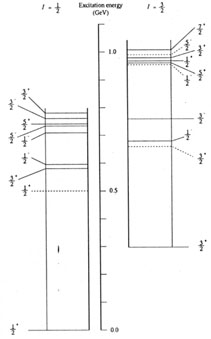Figure 1.2. An energy-level diagram for the nucleon and its excited states. The levels fall into two classes, isotopic doublets (I = 1/2) and isotopic quartets (I = 3/2). The states are labelled by their total angular momenta and parities Jp. The nucleon doublet N(939) is the ground state of the system the(1232) is the lowest lying quartet. Within the quark model (see text) these two states are the lowest that can be formed with no quark orbital angular momentum (L = 0). The other states designated by unbroken lines have clear interpretations: they are all the next most simple states with L = 1 (negative parity) and L = 2 (positive parity). The broken lines show states that have no clear interpretation within the simple three-quark model. They are perhaps associated with excited states of the gluon feds.

Energy level diagrams of the I = 3/2 and I = 1/2 states up to excitation energies of 1 GeV are shown in Fig. 1.2. The energy differences between states in a multiplet are only of the order of 1 MeV and cannot be shown on the scale of the figure. The widthsof the excited states are however quite large, of the order of 100 MeV, corresponding to mean lives=/~ 10-23 s. The excited states are all energetic enough to decay through the strong interaction, as for example++ -> p ++ (Fig. 1.3).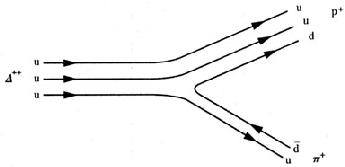Figure 1.3. A quark model diagram of the decay++ -> p ++. The gluon field is not represented in this diagram, but it would be responsible for holding the quark systems together and for the creation of the d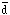pair.

 Quark Isospinl I3 u 1/2 1/2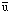1/2 -1/2 d 1/2 -1/21/2 1/2 s 0 0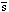0 0

Mesons made up of light u and d quarks and their antiquarks also have a rich spectrum of states which can be classified by their isospin. Antiquarks have an I3 of opposite sign to that of their corresponding quark (Table 1.4). By the rules for the addition of isospin, quark-antiquark pairs have I = 0 or I = 1. The I = 0 states are singlets with charge 0, like the(Fig. 1.4(a)). The I = 1 states make up triplets carrying charge +e, 0, -e, which are almost degenerate in energy, like the triplet+,0,-.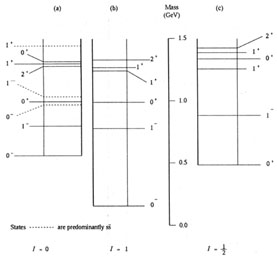Figure 1.4. States of the quark-antiquark system u, u, d, dform isotopic triplets (I = 1): u, (u- d) /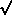2, d; and also isotopic singlets (I = 0): (u+ d) /2. Figure 1.4(a) is an energy-level diagram of the lowest energy isosinglets, including states -- which are interpreted as sstates. Figure 1.4(b) is an energy-level diagram of the lowest energy isotriplets. Figure 1.4(c) is an energy-level diagram of the lowest energy K mesons. The K mesons are quark-antiquark systems uand d; they are isotopic doublets, as are their antiparticle states sand s. Their higher energies relative to the states in Fig. 1.4(b) are largely due to the higher mass of the s over the u and d quarks. The large relative displacement of the 0+ state is a feature with, as yet, no clear interpretation.

The spectrum of I = 1 states with energies up to 1.5 GeV is shown in Fig. 1.4(b). As in the baryon case the splitting between states in the same isotopic multiplet is only a few MeV; the widths of the excited states are like the widths of the excited baryon states, of the order of 100 MeV. In the lowest multiplet (the pions), the quark-antiquark pair is in an L = 0 state with spins coupled to zero. Hence Jp = 0-, since a fermion and antifermion have opposite relative parity (Section 6.4). In the first excited state the spins are coupled to 1 and Jp = 1-. These are the p mesons. With L = 1 and spins coupled to S = 1 one can construct states 2+, 1+, 0+, and with L = 1 and spins coupled to S = 0 a state 1+. All these states can be identified in Fig. 1.4(b).